Ex 14.3

Chapter 14 Class 8 Factorisation
Serial order wise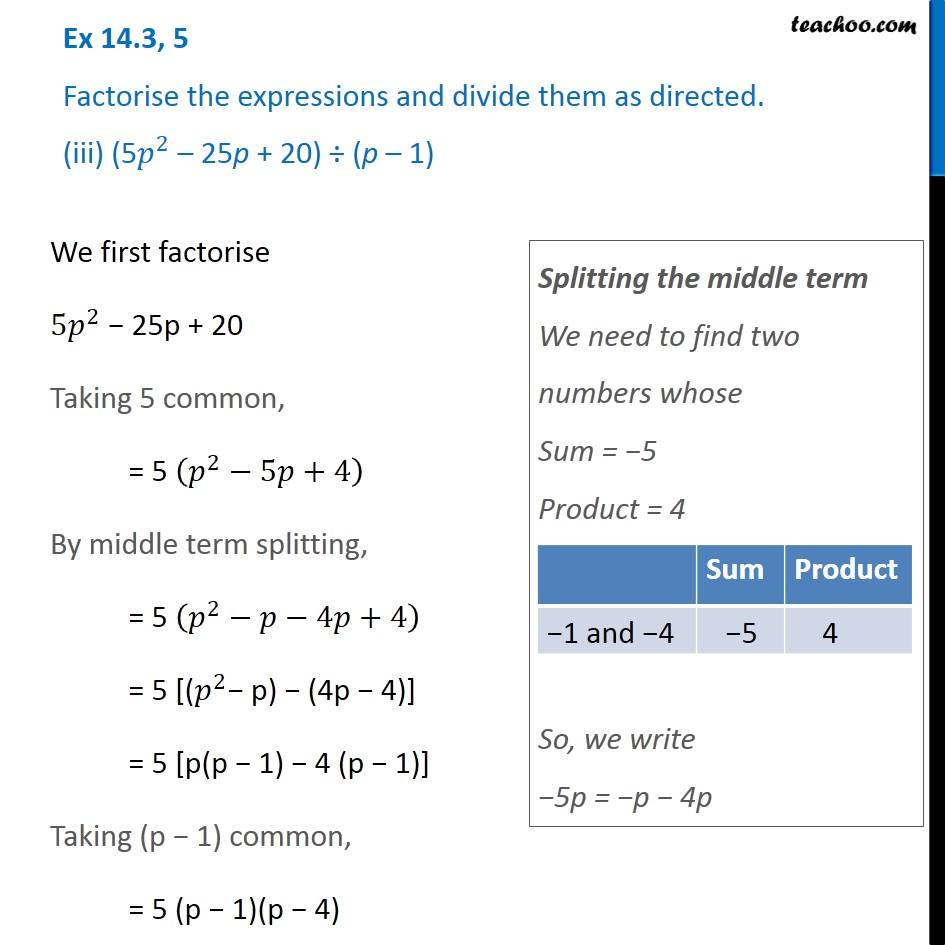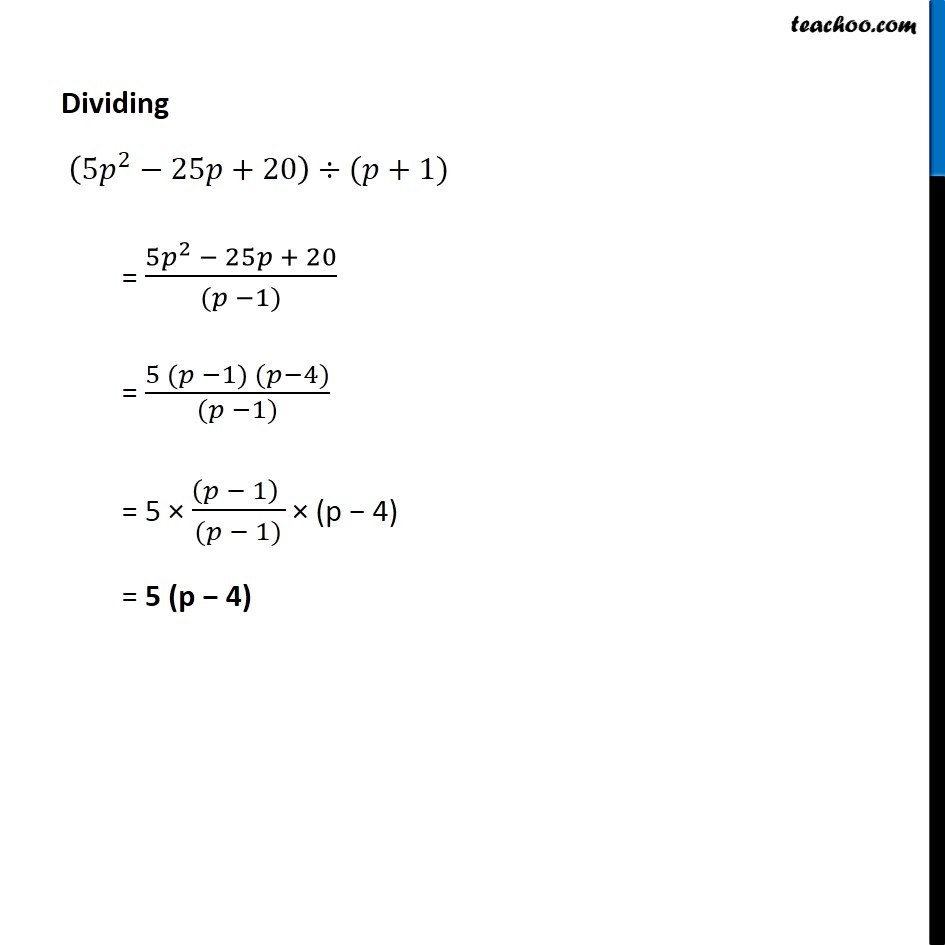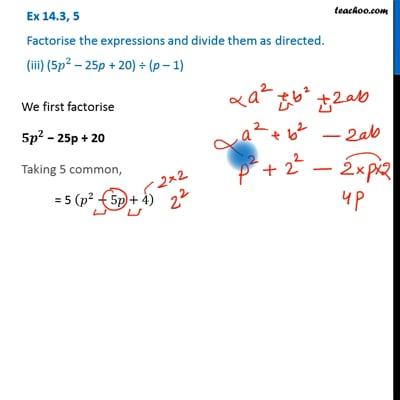This video is only available for Teachoo black users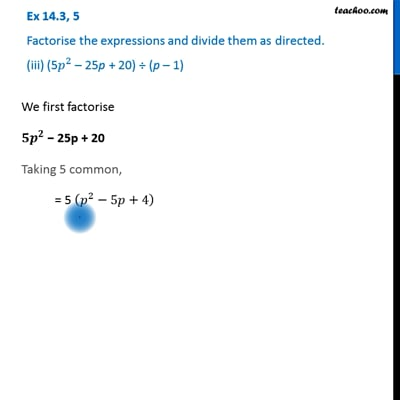This video is only available for Teachoo black users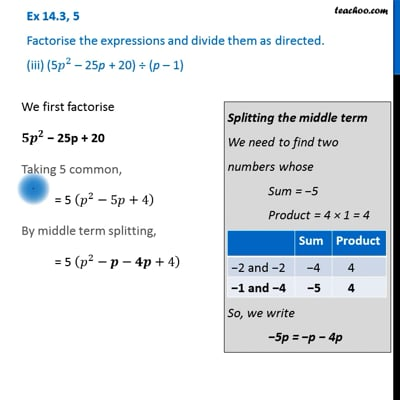This video is only available for Teachoo black users

Get live Maths 1-on-1 Classs - Class 6 to 12

### Transcript

Ex 14.3, 5 Factorise the expressions and divide them as directed. (iii) (5𝑝^2 – 25p + 20) ÷ (p – 1) We first factorise 〖5𝑝〗^2 − 25p + 20 Taking 5 common, = 5 (𝑝^2−5𝑝+4) By middle term splitting, = 5 (𝑝^2−𝑝−4𝑝+4) = 5 [(𝑝^2− p) − (4p − 4)] = 5 [p(p − 1) − 4 (p − 1)] Taking (p − 1) common, = 5 (p − 1)(p − 4) Splitting the middle term We need to find two numbers whose Sum = −5 Product = 4 So, we write −5p = −p − 4p Dividing (〖5𝑝〗^2−25𝑝+20)÷(𝑝+1) = (〖5𝑝〗^2 − 25𝑝 + 20)/((𝑝 −1)) = (5 (𝑝 −1) (𝑝−4))/((𝑝 −1)) = 5 × ((𝑝 − 1) )/((𝑝 − 1)) × (p − 4) = 5 (p − 4)